# Online Math Games for High School Students

Starting with Algebra I, it gets harder and harder to find good online math games and programs to help high school students practice and study. And that’s unfortunate since high school students are usually the ones who need the most help. While most of the online games available are focused on the more basic skills, there are a few that work with higher level concepts like factoring. Here are the best free online math programs that I’ve found that might be helpful practice for high school students, starting with general games first and moving on to games aimed at specific math topics.

# Free Online Math Games for Algebra

#### The X Detective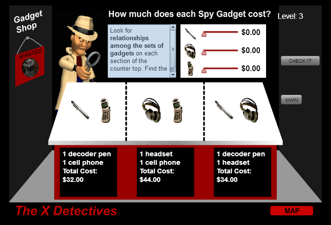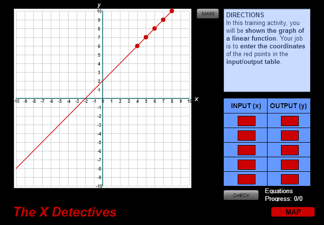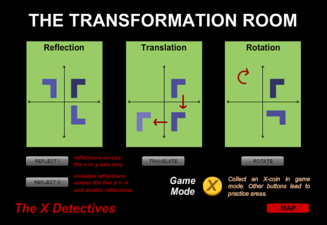The X Detectives covers a variety of math topics. Under the guise of a 3rd person role-playing scenario, this game leads students through several games that teach algebraic reasoning, transformations, and graphing linear equations (functions). It’s a good resource for refreshing or learning basic Algebra skills.

#### Aptly named, this algebra game creates visual equations using scales. To play, students must solve for the value of each image (variable) and substitute it into the new equation created by the goal. This is good practice for solving equations and applying logic in Algebra.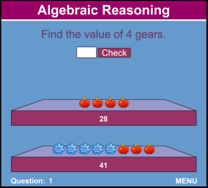#### Sum Shapes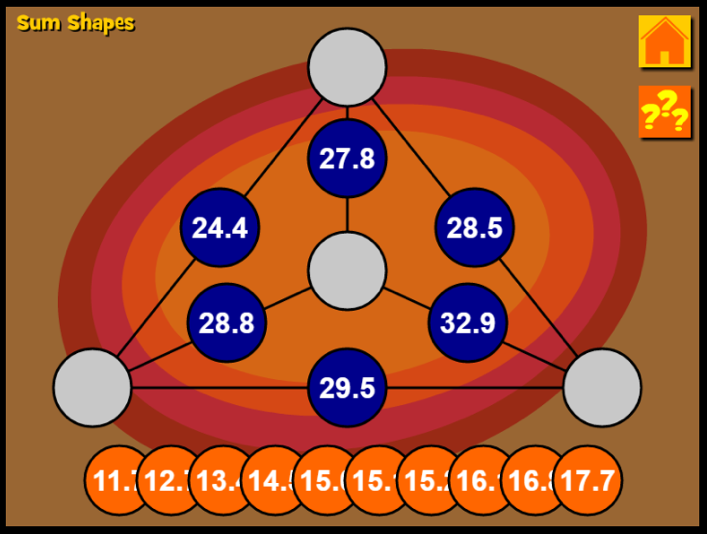Sum Shapes is a logic puzzle where students have to take numbers from the key and insert them into the shape so that all the existing numbers add along lines to form the numbers in the corners. It can be quite a challenge, so it’s great for building logic and reasoning skills!

#### Line Gem 1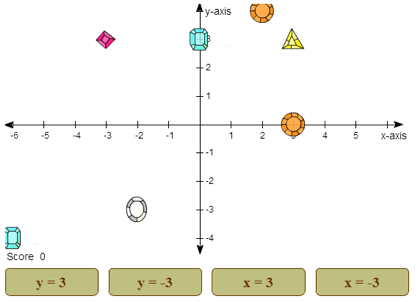This game mixes strategy with graphing in slope-intercept form. Students pick the equation of a line and earn points for each gem collected on that line. Improved scores come from recognizing the equations of the different lines and picking the one with the most gems on it. Difficulty increases as the game goes on.

#### Algebra vs. The Cockroaches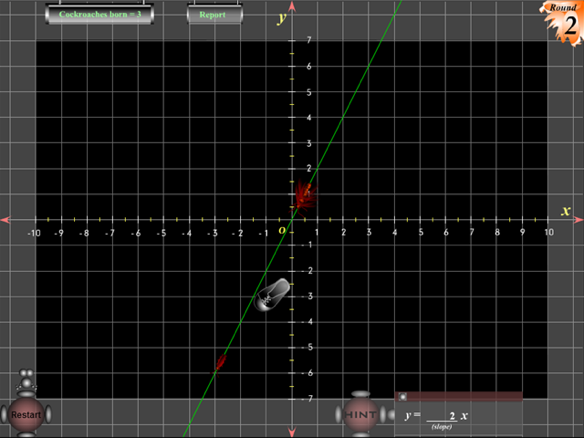Take away a little of Line Gem 1’s strategy and toss in a thrown shoe, and you get Algebra vs. The Cockroaches. In this online math game, students have to fill in the slope or the y-intercept of the equation for the line that the cockroaches are walking on. The longer that takes, the more cockroaches that will be born. Completing the correct line fires the weapon of the student’s choice (such as a shoe) and destroys the cockroaches.

Unearthworms

####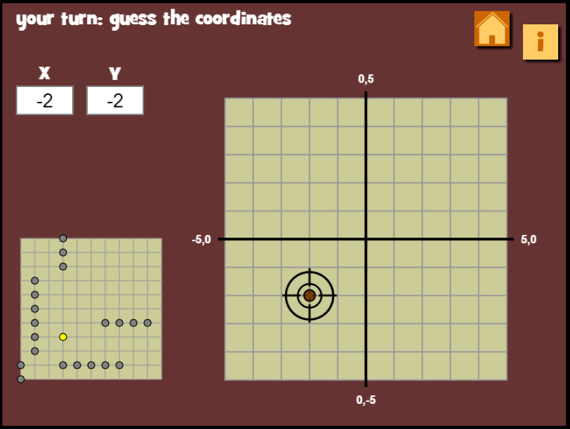Unearthworms is a multiplayer math game on a coordinate grid where students can play against another or the computer. Like Battleship, players hide their earthworms and then picks points on the coordinate grid to guess where other players’ earthworms might be. If students need practice plotting points, this is the game for them.

# Online Math Programs to Help with Factoring, Exponents, & Square Roots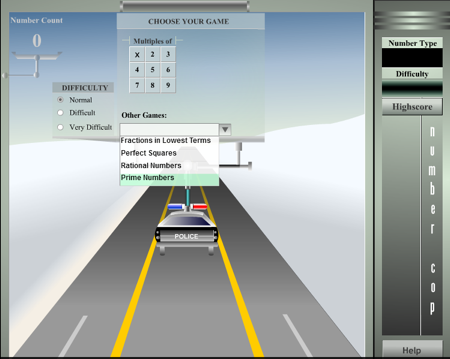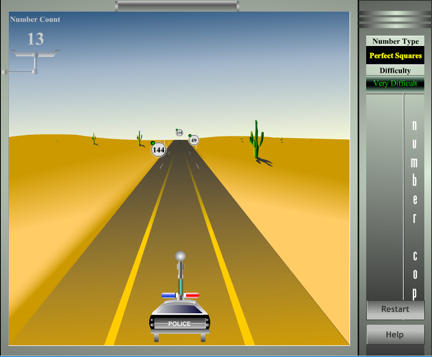This game is ranked number 1 in this section for several reasons. First, you can pick the topic (perfect squares, rational numbers, etc.). Second, you can pick the difficulty level, which makes it better practice over time. Last but not least, it’s actually an interactive game where you have to drive the police car to pick up the target numbers.